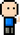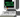# Josh Meets Computer# Find Nearest Number

May 31, 2018

This write-up solves the following algorithm challenge:

“Given a value X and a series of numbers, find the nearest number to X”

A good way to solve an algorithm challenge is to start with a simple, digestible example.

``````import numpy as np

X = 52
series = np.random.choice(np.arange(100), 15) ``````
``series``
``array([96, 63, 71, 61, 71, 21, 42, 19, 33, 14, 71, 83, 74, 42, 50])``

One way to tackle this faster than checking the distance between X and every value in the series is to take the array of data and restructure it as a binary tree. From there a binary search can find the value in O(log(N)) time. The worst case scenario being the series value is the lowest value in the series, so Binary Search would search every layer until it got to the smallest leaf.

``````class node():

def __init__(self, value):
self.value = value
self.left  = None
self.right = None

def __call__(self):
return self.value

class tree():

def __init__(self):
self.root = None

if len(values) > 1:
for v in values:
else:

if self.root is None:
self.root = node(value)
else:

if value < curr_node.value:
if curr_node.left is None:
curr_node.left = node(value)
else:
else:
if curr_node.right is None:
curr_node.right = node(value)
else:

def find(self, value):
if value < self.root.value:
if self.root.left is None:
return self.root.value
else:
return self._find(self.root.left, value)
elif value > self.root.value:
if self.root.right is None:
return self.root.value
else:
return self._find(self.root.right, value)
else:
return self.root.value

def _find(self, curr_node, value):
if value < curr_node.value:
if curr_node.left is None:
return curr_node.value
else:
return self._find(curr_node.left, value)
elif value > curr_node.value:
if curr_node.right is None:
return curr_node.value
else:
return self._find(curr_node.right, value)
else:
return curr_node.value``````
``````T = tree()
``Closest number to 52 is: 50``